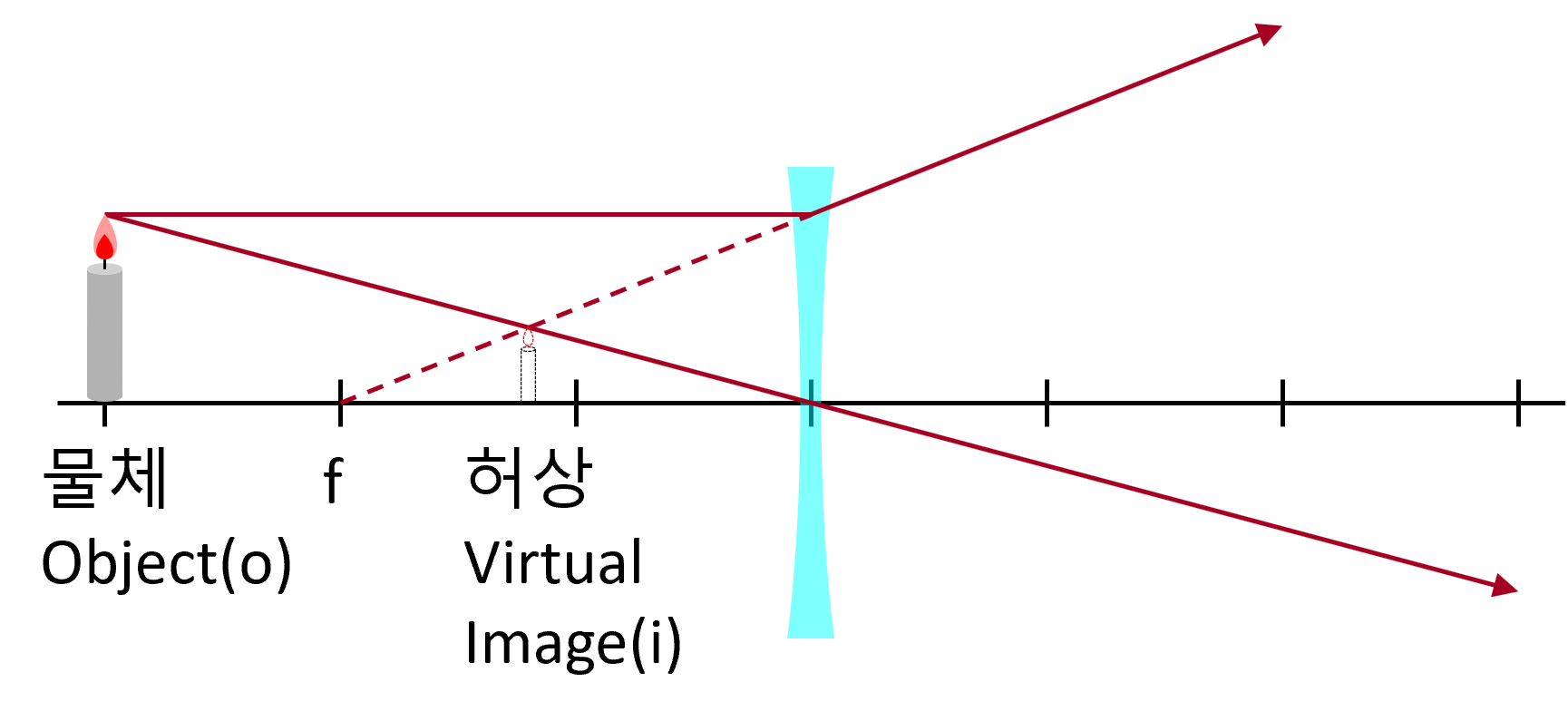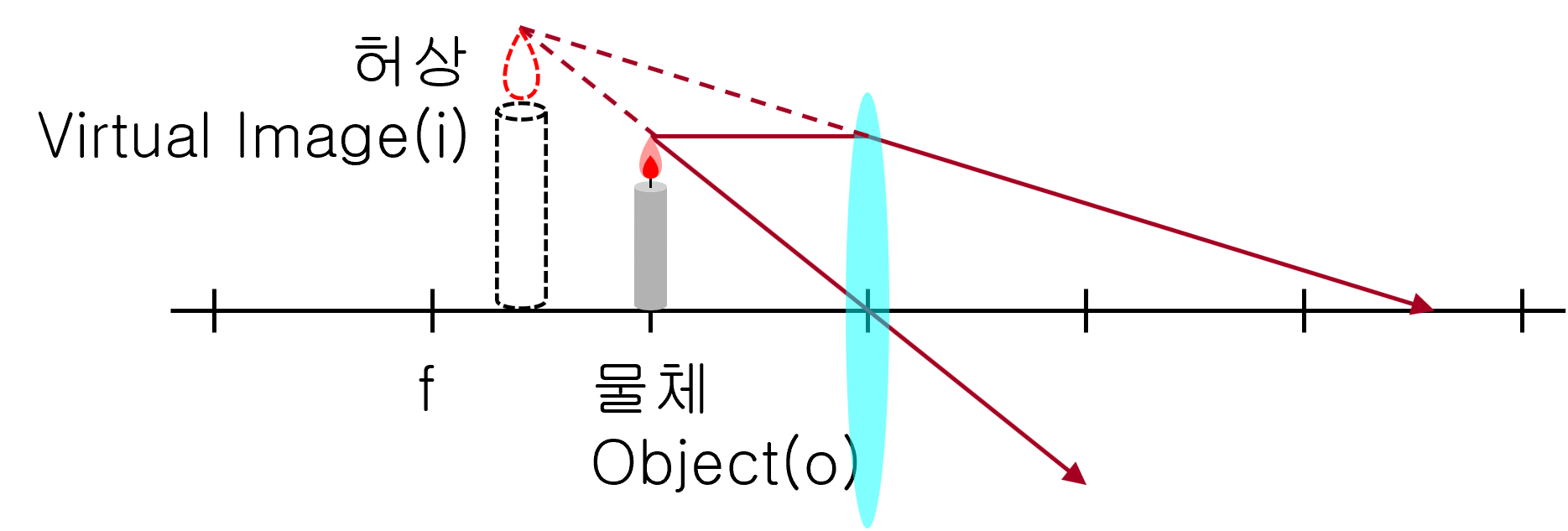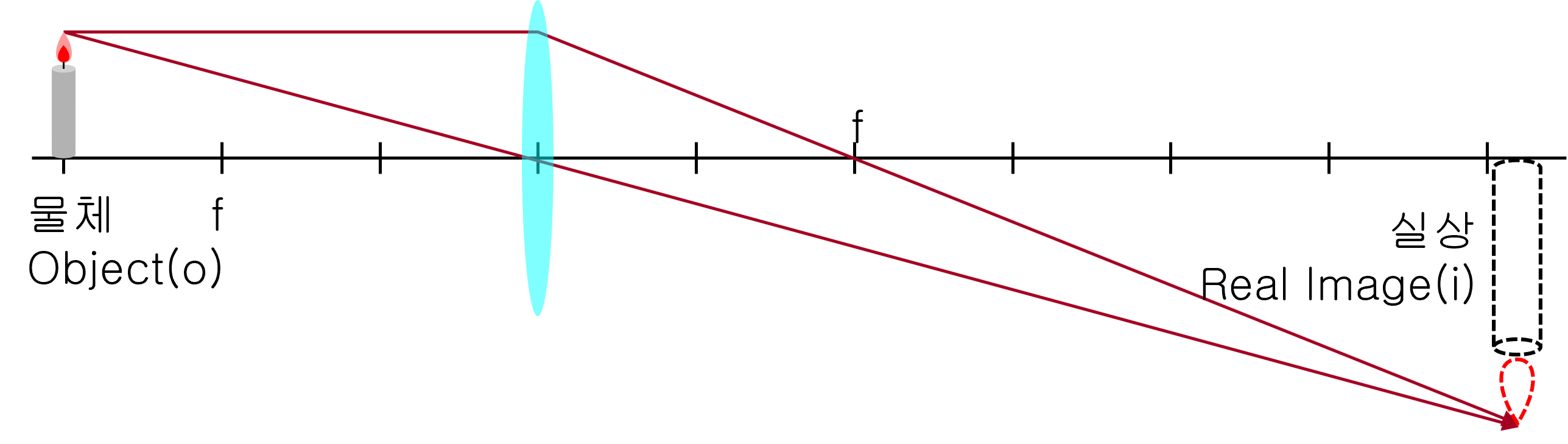# Convex Lens & Concave Lens

## Real image and virtual image

Looking at an object, we feel there is an object in it. By the way, if you feel that there is something, we say there is an ‘image,’ even if there is no real object or emitter.
The ‘image’ in optics is caused by the refraction or reflection of light.

Real image: Indicates that the light is actually gathered there and then starts again. If you put the paper in the position where the actual image is formed, you can actually see the image formed on the paper.

Virtual image: A case where there is no light at the place where ‘image’ is formed. If you put the paper in the position where the image is formed, no image appears.

## A virtual image of a concave lens

The image through the concave lens is always a virtual image, standing like an object, and looks smaller than the real thing.## Virtual image and real image of convex lens

The convex lens differs greatly depending on whether the object is inside the focus of the concave mirror.

Virtual image: If the object is inside the focus of the lens, the image is enlarged. And the image always stands straight.Real image: If the object is out of focus of the lens, it will be enlarged or reduced according to the distance from the lens. And always upside down.## Getting the distance of the image

The following simple formula is established between the distance of the object from the lens ‘p,’ the distance of the image from the lens ‘i’, and the focal length of the lens ‘f.’

$\frac { 1 }{ o } +\frac { 1 }{ i } =\frac { 1 }{ f }$

• o: Distance from lens to object. It always has positive (+) value.
• i: Distance from lens to image
• f: Focal length

In the case of a concave lens,

• o > 0: Real object
• i < 0: Virtual image
• f < 0: Virtual focus

In the case of a convex lens,

• o > 0: Real object
• f > 0: Real focus
• i = ?: It depends on the situation.
• In the case of o < f : i < 0 (virtual image standing upright)
• In the case of o = f : i value can not be obtained.
• In the case of o > f : i < 0 (Inverted real image)

## Ray tracing method

If you trace the path of at least two rays, you can find the image.

1. A ray parallel to the central axis passes through the focus after refraction.
2. A ray that passes through the focus and enters the lens is refracted and travels parallel to the axis.
3. The rays incident on the center of the lens proceed without changing the path.

## Obtaining Magnification

The magnification of the lens is…

$m=-\frac { i }{ o }$

• m: magnification
• i: Distance from mirror to image
• o: Distance from mirror to object

Depending on the value of m calculated, the image looks like this:

• m > 0: The image stands straight.
• m < 0: The image is inverted.
• |m| > 1: The image is magnified.
• |m| < 1: The image is reduced.